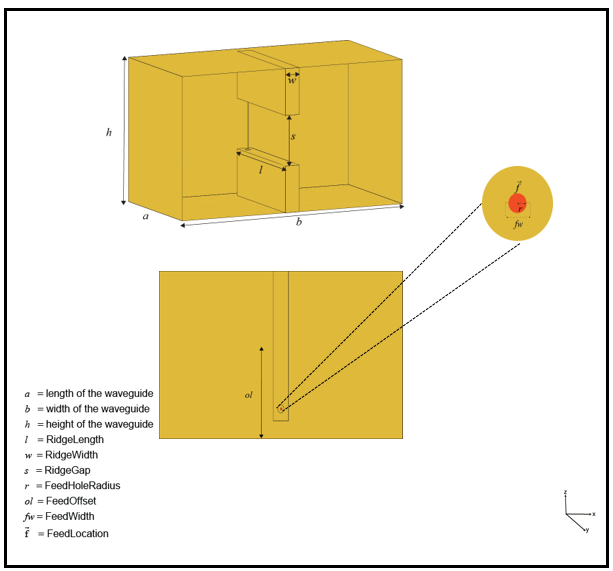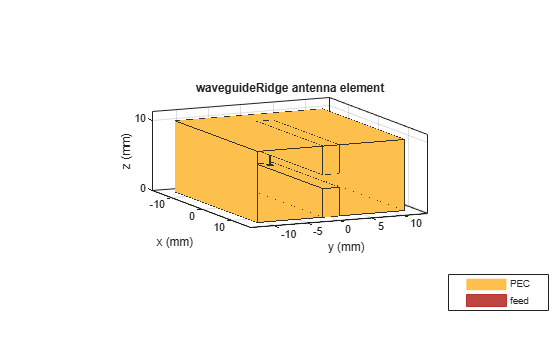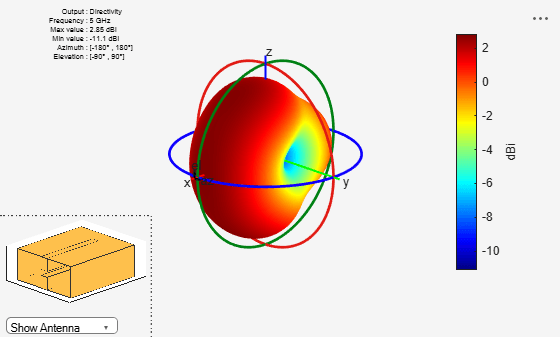Main Content

# waveguideRidge

Create ridged waveguide antenna

## Description

The `waveguideRidge` object creates a dual ridged waveguide antenna. The ridges ensure a smooth transition from an input impedance of 50 ohms to the impedance of free space (377 ohms). The dual ridged waveguide antenna widely used in ultra-wideband applications covering a large spectrum of frequencies. Ridged waveguide antennas are used in radio astronomy applications.## Creation

### Syntax

``ant = waveguideRidge``
``ant = waveguideRidge(Name,Value)``

### Description

example

````ant = waveguideRidge` creates a double-ridged waveguide antenna. The default `waveguideRidge` antenna object is centered on the XY-plane. The object dimensions are chosen for an operating frequency of 8-10 GHz.```

example

````ant = waveguideRidge(Name,Value)` sets properties using one or more name-value pairs. For example, `ant = waveguideRidge('Height',1)` creates a ridge waveguide with a height of 1 meter.```

Note

Properties you do not specify retain their default values.

## Properties

expand all

Length of the waveguide, specified as a real-valued scalar in meters.

Example: `'Length',0.0410`

Example: `ant.Length = 0.0410`

Data Types: `double`

Width of the waveguide, specified as a real-valued scalar in meters.

Example: `'Width',0.0640`

Example: `ant.Width = 0.0640`

Data Types: `double`

Height of the waveguide, specified as a real-valued scalar in meters.

Example: `'Height',0.0340`

Example: `ant.Height = 0.0340`

Data Types: `double`

Length of the ridge, specified as a real-valued scalar in meters.

Example: `'RidgeLength',0.0220`

Example: `ant.RidgeLength = 0.0220`

Data Types: `double`

Width of the ridge, specified as a real-valued scalar in meters.

Example: `'RidgeWidth',0.0030`

Example: `ant.RidgeLength = 0.0060`

Data Types: `double`

Gap between two ridges, specified as a real-valued scalar in meters.

Example: `'RidgeGap',0.0070`

Example: `ant.RidgeGap = 0.0098`

Data Types: `double`

Radius of the feeding hole, specified as a real-valued scalar in meters.

Example: `'FeedHoleRadius',0.00006`

Example: `ant.FeedHoleRadius = 0.00010`

Data Types: `double`

Width of the feed, specified as a real-valued scalar in meters.

Example: `'FeedWidth',0.0010`

Example: `ant.FeedWidth = 0.0020`

Data Types: `double`

Signed distances from the origin measured along the length and width of the waveguide, specified as a two-element vector with each element in meters.

Example: `'FeedOffset',[-0.0725 0]`

Example: `ant.FeedOffset = [-0.0830 0]`

Data Types: `double`

Type of the metal used as a conductor, specified as a metal material object. You can choose any metal from the `MetalCatalog` or specify a metal of your choice. For more information, see `metal`. For more information on metal conductor meshing, see Meshing.

Example: ```m = metal('Copper'); 'Conductor',m```

Example: ```m = metal('Copper'); ant.Conductor = m```

Lumped elements added to the antenna feed, specified as `lumpedelement ` object. You can add a load anywhere on the surface of the antenna. By default, the load is at the feed. For more information, see `lumpedElement`.

Example: `'Load',lumpedelement`. The`lumpedelement` is a object handle for the load created using `lumpedElement`.

Example: ```ant.Load = lumpedElement('Impedance',75)```

Tilt angle of the antenna, specified as a scalar or vector with each element unit in degrees. For more information, see Rotate Antennas and Arrays.

Example: `'Tilt',90`

Example: `ant.Tilt = 90`

Example: `'Tilt',[90 90]`,`'TiltAxis',[0 1 0;0 1 1]` tilts the antenna at 90 degrees about the two axes defined by the vectors.

Note

The `wireStack` antenna object only accepts the dot method to change its properties.

Data Types: `double`

Tilt axis of the antenna, specified as:

• Three-element vector of Cartesian coordinates in meters. In this case, each coordinate in the vector starts at the origin and lies along the specified points on the X-, Y-, and Z-axes.

• Two points in space, each specified as three-element vectors of Cartesian coordinates. In this case, the antenna rotates around the line joining the two points in space.

• A string input describing simple rotations around one of the principal axes, 'X', 'Y', or 'Z'.

For more information, see Rotate Antennas and Arrays.

Example: `'TiltAxis',[0 1 0]`

Example: `'TiltAxis',[0 0 0;0 1 0]`

Example: `ant.TiltAxis = 'Z'`

Note

The `wireStack` antenna object only accepts the dot method to change its properties.

Data Types: `double`

## Object Functions

 `show` Display antenna or array structure; display shape as filled patch `impedance` Input impedance of antenna; scan impedance of array `sparameters` S-parameter object `returnLoss` Return loss of antenna; scan return loss of array `vswr` Voltage standing wave ratio of antenna `optimize` Optimize antenna or array using SADEA optimizer `pattern` Radiation pattern and phase of antenna or array; Embedded pattern of antenna element in array `patternAzimuth` Azimuth pattern of antenna or array `patternElevation` Elevation pattern of antenna or array `axialRatio` Axial ratio of antenna `beamwidth` Beamwidth of antenna `charge` Charge distribution on metal or dielectric antenna or array surface `current` Current distribution on metal or dielectric antenna or array surface `design` Design prototype antenna or arrays for resonance at specified frequency `efficiency` Radiation efficiency of antenna `EHfields` Electric and magnetic fields of antennas; Embedded electric and magnetic fields of antenna element in arrays `mesh` Mesh properties of metal or dielectric antenna or array structure

## Examples

collapse all

Create` a waveguideRidge antenna` object with default properties.

`a = waveguideRidge`
```a = waveguideRidge with properties: Length: 0.0210 Width: 0.0400 Height: 0.0250 RidgeLength: 0.0187 RidgeWidth: 0.0025 RidgeGap: 0.0088 FeedHoleRadius: 5.0000e-04 FeedWidth: 1.0000e-04 FeedOffset: [-0.0067 0] Conductor: [1x1 metal] Tilt: 0 TiltAxis: [1 0 0] Load: [1x1 lumpedElement] ```

Create a `waveguideRidge` antenna object with the properties specified.

```h = waveguideRidge('Length',0.0273,'Width', 0.02286,'RidgeGap',2e-3, ... 'Height', 0.01016,'RidgeLength',0.022);```

View the `waveguideRidge` antenna using a `show` function.

```figure show(h) ```Plot the 3-D radiation pattern of the `waveguideRidge` antenna at 5 GHz.

`pattern(h,5e9)`## See Also

### Topics

Introduced in R2019b

## Support

#### Hybrid Beamforming for Massive MIMO Phased Array Systems

Download the white paper